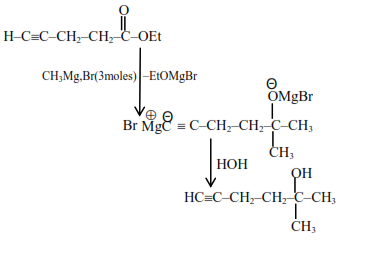# Given below are two statements :`
Question:

Given below are two statements :

Statement I : Ethyl pent-4-yn-oate on reaction with $\mathrm{CH}_{3} \mathrm{MgBr}$ gives a $3^{\circ}$-alcohol.

Statement II : In this reaction one mole of ethyl pent-4-yn-oate utilizes two moles of $\mathrm{CH}_{3} \mathrm{MgBr}$.

In the light of the above statements, choose the most appropriate answer from the options given below :

1. Both Statement I and Statement II are false.

2.  Statement I is false but Statement II is true.

3.  Statement I is true but Statement II is false

4. Both Statement I and Statement II are true.

Correct Option: , 3

Solution:

Statement 1 is true

But it consume 3 moles of G R

So statement 2 is false.